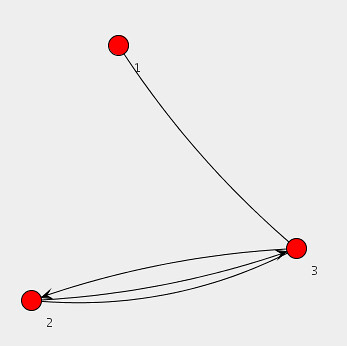# Java Universal Network/Graph Framework

Recently, I’m primarily concerned with large-scale graph data processing. Occasionally, the visualization of graph can be a good way for us to observe some properties from graph data sets. Today, I’m going to introduce a graph framework, called Java Universal Network/Graph Framework (Jung). Jung provides data structures for graph, a programming interface familiar with graph features, some fundamental graph algorithms (e.g., minimum spanning tree, depth-first search, breath-first search, and dijkstra algorithm), and even visualization methods. Especially, I’m interested in its visualization methods.

The following java source shows the programming interface of Jung. In more detail, this program make a graph, add three vertices to the graph, and connect vertices. This source code is brought from Jung tutorial. As you can see, Jung’s APIs are very easy.

```  // Make a graph by a SparseMultigraph instance.
Graph&lt;Integer, String&gt; g = new SparseMultigraph&lt;Integer, String&gt;();
g.addEdge(&quot;Edge-A&quot;, 1,3); // Added an edge to connect between 1 and 3 vertices.
g.addEdge(&quot;Edge-P&quot;, 2,3); // A parallel edge

// Make some objects for graph layout and visualization.
Layout&lt;Integer, String&gt; layout = new KKLayout&lt;Integer, String&gt;(g);
BasicVisualizationServer&lt;Integer, String&gt; vv =
new BasicVisualizationServer&lt;Integer, String&gt;(layout);
vv.setPreferredSize(new Dimension(800,800));

// It determine how each vertex with its value is represented in a diagram.
ToStringLabeller&lt;Integer&gt; vertexPaint = new ToStringLabeller&lt;Integer&gt;() {
public String transform(Integer i) {
return &quot;&quot;+i;
}
};

vv.getRenderContext().setVertexLabelTransformer(vertexPaint);

JFrame frame = new JFrame(&quot;Simple Graph View&quot;);
frame.setDefaultCloseOperation(JFrame.EXIT_ON_CLOSE);